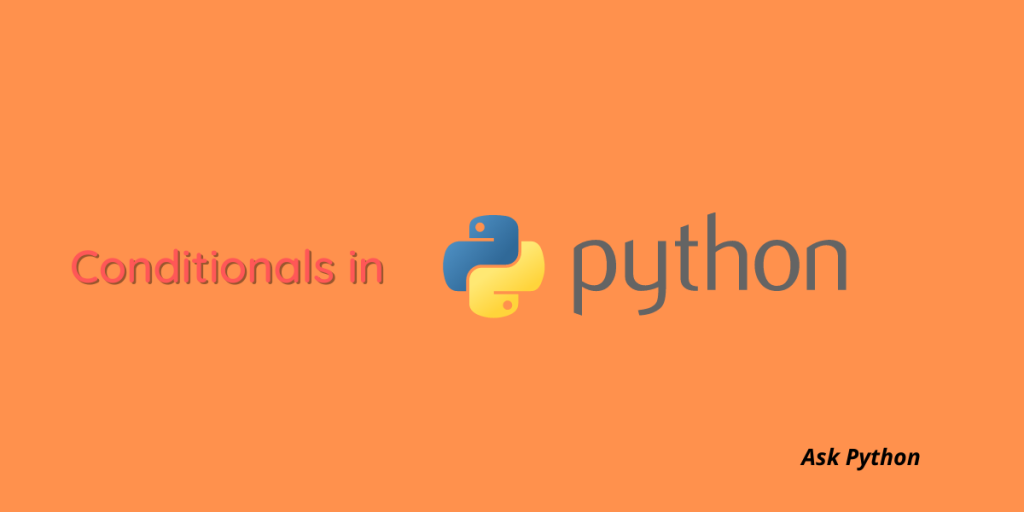# Conditionals in Python – A Quick GuideThe world is much more complex than it seems. That is why in some cases we need to put conditions to resolve the complexity. With the help of conditionals, you can skip over some complexities or run a series of statements very quickly. You can also choose between statements and execute a program. For instance, in everyday life, we use a lot of conditionals such as if it snows today, I will not go to the market or else I will go.

## Conditionals in Python

In this tutorial, we are going to learn how we can use conditionals in Python. So let’s get started!

Let’s look at the conditional statements one by one.

### If Statement

We will start with the very basic “if” statement. The syntax of the if statement is as follows:

```if(condition):
(statement)
```

The syntax means that if the condition satisfies, the statement will be executed, or else it will be skipped.

For example:

```A=1
B=3

if(A<B):
print(‘A is true’)

```

Output:

```A is true
```

Now, if we want to execute multiple statements together, the if statement will look something like this:

```if(condition):

<statement>
<statement>
<statement>
……………

< Other statements>

```

### Else Statement

Let’s now see what the else condition looks like. In this case, we will combine the if statement with the else condition.

The basic syntax of the else statement:

```if(condition):
<statement>

else:
<statement>

```

For example:

```x=200

If x<300:
print(‘200 is smaller’)

else:
print(‘200 is bigger’)

```

Output:

```200 is smaller
```

### Elif Statement

The elif statement is another conditional statement in Python that helps to check for multiple conditions that are true. Elif statement is almost like the if statement with the exception that there can only be one else statement whereas there can be multiple elif statements.

Following is the syntax of the elif statement.

```if(condition1):
<statement>

elif(condition2):
<statement>
elif(condition3):
<statement>
else:
<statement>

```

Let’s take a look at the following example.

```price=180

if price>100:
print(“Price is greater than 100”)

elif price==100:
print(“Price is 100”)

elif price<100:
print(“Price is less than 100”)

else :
print(“Invalid Price”)

```

Output:

```Price is greater than 100
```

Note: All the statements should maintain their indentation level or else it will throw an indentation error.

## Conclusion:

In this article, we have learned about the conditionals or control structures in Python. These control structures determine the execution of a program. You can use loops along with these control structures to execute different programs. Hence, it is very important for you to know the conditional statements in Python.